Die and Wafer
Diodes and Rectifiers
Discrete Thyristors
IC - Power and Linear
MOSFETs
Optoelectronics
Power Modules
Capacitors
Resistors
Magnetics
Sensors
Other Components
Semiconductors
Passive Components
 My Vishay | Language

# Small Signal Diodes - Small signal switching diodes - Repetitive Peak Reverse Voltage above 100 V

Small Signal Diodes - Small signal switching diodes - Repetitive Peak Reverse Voltage above 100 V
Series
Product ImagePackage (JEDEC®) Code
VRRM
(V)
IR at VR
(nA at V)
VF at IF
(V at mA)
trr
(ns)
Circuit Configuration
AEC-Q101 Qualified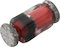Enlarge
QuadroMELF (SOD-80) 140 1 at 60 1 at 100 n/a Single No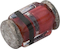Enlarge
MicroMELF 140 1 at 60 1 at 100 0 Single No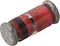Enlarge
MiniMELF (SOD-80) 140 1 at 60 1 at 100 0 Single No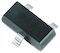Enlarge
SOT-23 120 100 at 100 1.0 at 100 50 Single Yes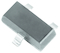Enlarge
SOT-23 120 100 at 100 1.0 at 100 50 Single YesEnlarge
SOT-23 200 100 at 150 1.0 at 100 50 Single YesEnlarge
SOT-23 200 100 at 150 1.0 at 100 50 Single YesEnlarge
SOT-23 250 100 at 200 1.0 at 100 50 Single YesEnlarge
SOT-23 250 100 at 200 1.0 at 100 50 Single Yes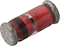Enlarge
MiniMELF (SOD-80) 120 100 at 100 1 at 100 50 Single YesEnlarge
MiniMELF (SOD-80) 200 100 at 150 1 at 100 50 Single YesEnlarge
MiniMELF (SOD-80) 250 100 at 200 1 at 100 50 Single YesEnlarge
DO-35 (DO-204AH) 120 100 at 100 1 at 100 50 Single Yes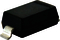Enlarge
SOD-123 120 100 at 100 1 at 100 50 Single Yes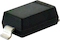Enlarge
SOD-123 120 100 at 100 1 at 100 50 Single Yes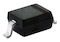Enlarge
SOD-323 120 100 at 100 1 at 100 50 Single YesEnlarge
SOD-323 120 100 at 100 1 at 100 50 Single YesEnlarge
DO-35 (DO-204AH) 200 100 at 150 1 at 100 50 Single Yes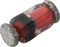Enlarge
QuadroMELF (SOD-80) 120 100 at 100 1 at 100 50 Single NoEnlarge
QuadroMELF (SOD-80) 200 100 at 150 1 at 100 50 Single NoEnlarge
QuadroMELF (SOD-80) 250 100 at 200 1 at 100 50 Single NoEnlarge
SOD-123 200 100 at 150 1 at 100 50 Single YesEnlarge
SOD-123 200 100 at 150 1 at 100 50 Single YesEnlarge
SOD-323 200 100 at 150 1 at 100 50 Single YesEnlarge
SOD-323 200 100 at 150 1 at 100 50 Single YesEnlarge
DO-35 (DO-204AH) 250 100 at 200 1 at 100 50 Single YesEnlarge
SOD-123 250 100 at 200 1 at 100 50 Single YesEnlarge
SOD-123 250 100 at 200 1 at 100 50 Single YesEnlarge
SOD-323 250 100 at 200 1 at 100 50 Single YesEnlarge
SOD-323 250 100 at 200 1 at 100 50 Single YesEnlarge
SOT-23 250 100 at 200 1 at 100 50 Common cathode YesEnlarge
SOT-23 250 100 at 200 1 at 100 50 Common cathode Yes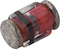Enlarge
MicroMELF 120 100 at 100 1 at 100 50 Single YesEnlarge
MicroMELF 200 100 at 150 1 at 100 50 Single YesEnlarge
MicroMELF 250 100 at 200 1 at 100 50 Single YesEnlarge
DO-35 (DO-204AH) 140 3 at 60 1 at 30 n/a Single NoEnlarge
SOT-23 300 100 at 240 0.87 at 20 50 Common anode YesEnlarge
SOT-23 300 100 at 240 0.87 at 20 50 Common anode YesEnlarge
SOT-23 300 100 at 240 0.87 at 20 50 Common cathode YesEnlarge
SOT-23 300 100 at 240 0.87 at 20 50 Common cathode YesEnlarge
SOT-23 300 100 at 240 0.87 at 20 50 Dual serial YesEnlarge
SOT-23 300 100 at 240 0.87 at 20 50 Dual serial YesEnlarge
SOD-123 300 100 at 240 1 at 100 50 Single YesEnlarge
SOD-123 300 100 at 240 1 at 100 50 Single Yes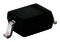Enlarge
SOD-323 300 100 at 240 0.87 at 20 50 Single YesEnlarge
SOD-323 300 100 at 240 0.87 at 20 50 Single Yes